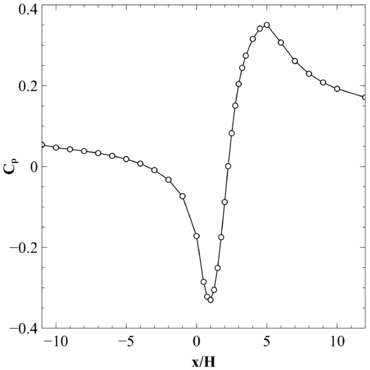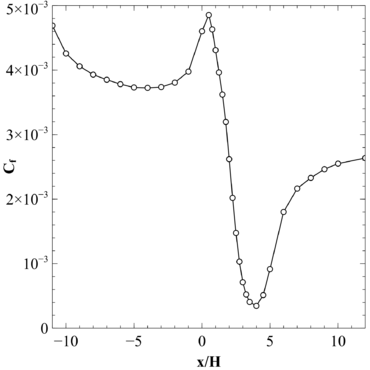# Statistical data

In this section the relevant statistical data for the flow on the smooth bump computed with MIGALE is given. The reported data is the one mentioned in Table 1 of the list of desirable quantities (PDF).

The data is available as:

• In .vtk (ASCII) format as statistical data (mid-span plane).
• In .vtu (ASCII) format for instantaneous data.
• In .csv (text) format as vertical profiles at various x-positions.

## Midplane data

Data of the statistics computed at the midspan of the computational domain are provided here. Statistics at the midspan are virtually the same for any other parallel plane. For more information regarding the stored quantities and the storage format, please refer to the storage format guidelines.

The available files are:

## Profile data

Profile data have been extracted at different streamwise locations ($x/H=-11,-10,-9,-8,-7,-6,-5,-4,-3,-2,-1,-0,0.5,0.75,1,1.25,1.5,1.75,2,2.25,2.5,2.75,3,3.25,3.5,4,4.5,5,6,7,8,9,10,12,14$) and made dimensionless with respect to reference quantities ($u_{ref}$, $\rho _{ref}$, $p_{s,ref}$, see UFR 3-36: Table 2). The data stored in each file are:

• streamwise location $x/H$• vertical location $y/H$• wall distance $(y-y_{wall})/H$• average pressure $p/p_{s,ref}$• average velocity components $U_{i}/u_{ref}$• Reynolds stress components $R_{ij}/(\rho _{ref}u_{ref}^{2})$• turbulent kinetic energy $k/u_{ref}^{2}$Profiles at selected streamwise locations are reported in Fig. 11.

## Contour data

Contour data of averaged velocity components and Reynolds stresses is also provided in Fig. 12. Reynolds stresses show an intensity peak which moves away from the wall as the flow moves above the rounded step. Downstream the step, however, such peak gets closer to the wall as the boundary layer is recovering the canonical behaviour for a zero pressure gradient configuration.

The following averaged quantities are provided along the solid wall:

• the pressure coefficient $C_{p}$• the skin friction coefficient $C_{f}$The pressure coefficient is defined as $C_{p}=2(p-p_{chk})/(\rho _{ref}u_{ref}^{2})$, where $p_{chk}$is the pressure at wall at the checkpoint $x/H=-3.5$, while the skin friction coefficient is defined as $C_{f}=2(\tau _{wall})/(\rho _{ref}u_{ref}^{2})$. $u_{ref}$and $\rho _{ref}$are the velocity and density at the reference conditions (see UFR 3-36: Table 2), respectively. Both coefficients have been obtained by averaging along the span direction the time averaged solution.

 Figure 13: HiFi-TURB-DLR rounded step, pressure and skin friction coefficients on the solid wallContributed by: Francesco Bassi, Alessandro Colombo, Francesco Carlo Massa — Università degli studi di Bergamo (UniBG)